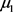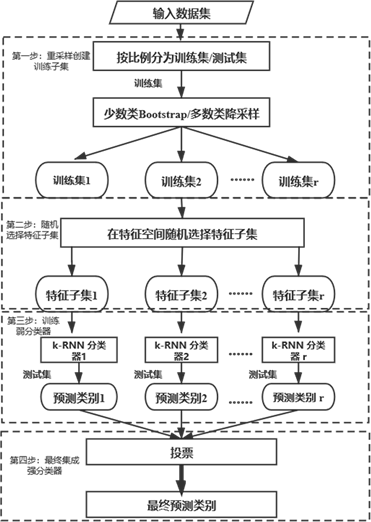#### 期刊菜单

An Ensemble Imbalanced Data Classification Algorithm Based on Random k-Rank Nearest Neighbor Rules
DOI: 10.12677/AAM.2020.95074, PDF, HTML, XML, 下载: 658  浏览: 814

Abstract: In this article, a random ensemble k-RNN algorithm called REKRNN is proposed to deal with the imbalanced data classification. The algorithm incorporates the k-rank nearest neighbor classifier into the frame of Bagging algorithm. At the same time, resampling techniques and random feature method are applied to deal with the imbalanced issue. We observe that the proposed method per-formed remarkably well on different imbalanced dataset. The random ensemble k-RNN algorithm can be considered as a promising tool for imbalanced classification.

1. 引言

2. 相关研究

2.1. 不平衡数据分类的集成学习算法

2.2. k-RNN分类器

$\left\{{X}_{1},{X}_{2},\cdots ,{X}_{{n}_{1}}\right\}$$\left\{{Y}_{1},{Y}_{2},\cdots ,{Y}_{{n}_{2}}\right\}$ 是分别来自两个给定总体 ${\pi }_{1}$${\pi }_{2}$ 的训练样本集，测试样例Z可被分类至总体 ${\pi }_{1}$${\pi }_{2}$。将X,Y和Z全部样本( ${n}_{1}+{n}_{2}+1$ 个)按照降序(或升序)排列，得到混合样本的秩次；然后，取Z左边的样本和右边的样本各k个作为秩次最近邻，样例Z的预测类别将由这2k个最近邻中的出现次数较多的类别标记为预测结果。

$\left\{{X}_{1},{X}_{2},\cdots ,{X}_{{n}_{1}}\right\}$$\left\{{Y}_{1},{Y}_{2},\cdots ,{Y}_{{n}_{2}}\right\}$ 是分别来自两个给定总体 ${\pi }_{1}$${\pi }_{2}$ 的训练样本集。其中 ${X}_{i}=\left({x}_{i1},{x}_{i2},\cdots ,{x}_{ip}\right)\in {R}^{p}$，总体均值为，协方差矩阵为 ${\Sigma }_{1}$${Y}_{i}=\left({y}_{i1},{y}_{i2},\cdots ,{y}_{ip}\right)\in {R}^{p}$，总体均值为 ${\mu }_{2}$，协方差矩阵为 ${\Sigma }_{2}$。测试样例 $Z=\left({z}_{1},{z}_{2},\cdots ,{z}_{p}\right)\in {R}^{p}$ 可被分类至总体 ${\pi }_{1}$${\pi }_{2}$。基于原始k-RNN规则，测试样例Z在多维样本X,Y和Z中的混合秩次由以下得分函数获得：

$R\left(Z;{\mu }_{1},{\mu }_{2},{\Sigma }_{1},{\Sigma }_{2}\right)=\left({\mu }_{1}^{\text{T}}{\text{Σ}}_{1}^{-1}-{\mu }_{2}^{\text{T}}{\text{Σ}}_{2}^{-1}\right)Z-\frac{1}{2}{Z}^{\text{T}}\left({\Sigma }_{1}^{-1}-{\Sigma }_{2}^{-1}\right)Z$ (1)

$\stackrel{^}{R}\left(Z;\stackrel{¯}{X},\stackrel{¯}{Y},{S}_{1},{S}_{2}\right)=\left({\stackrel{¯}{X}}^{\text{T}}{S}_{1}^{-1}-{\stackrel{¯}{Y}}^{\text{T}}{S}_{2}^{-1}\right)Z-\frac{1}{2}{Z}^{\text{T}}\left({S}_{1}^{-1}-{S}_{2}^{-1}\right)$ (2)

$\stackrel{¯}{X}=\frac{1}{{n}_{1}}{\sum }_{i=1}^{{n}_{1}}{X}_{i},\text{\hspace{0.17em}}\stackrel{¯}{Y}=\frac{1}{{n}_{2}}{\sum }_{i=1}^{{n}_{2}}{Y}_{i}$ (3)

${S}_{1}=\frac{1}{{n}_{1}-1}{\sum }_{i=1}^{{n}_{1}}\left({X}_{i}-\stackrel{¯}{X}\right){\left({Y}_{i}-\stackrel{¯}{Y}\right)}^{\text{T}},\text{\hspace{0.17em}}{S}_{2}=\frac{1}{{n}_{2}-1}{\sum }_{i=1}^{{n}_{2}}\left({X}_{i}-\stackrel{¯}{X}\right){\left({Y}_{i}-\stackrel{¯}{Y}\right)}^{\text{T}}$ (4)Table 1. k-RNN classifier algorithm

3. 随机秩次k-近邻集成学习算法

3.1. Bagging算法

Bagging是一种典型的集成学习算法，是当前机器学习算法研究的热点之一，在分类和回归任务中都有广泛的应用和良好的效果。它的实现步骤为：每次从训练集中有放回地抽取n个样本，重复抽取T次；由这T个样本各训练一个弱学习器；由T个弱学习器各对测试样本进行预测，按照投票取众数的方法得到最终预测结果。其中重要的步骤有放回抽样，即为Bootstrap抽样，Bagging名称的来源。它的主要思想是通过弱分类器之间的差异性提高模型泛化性能。针对不平衡数据分类的任务，REKRNN算法对Bagging框架做出如下改进：1) 采用混合重采样法对初始训练集进行采样以获得较为平衡的训练集；2) 引入随机特征子集降低维度和计算复杂度。

3.2. 混合重采样

3.3. 随机特征子集Table 2. Random feature space algorithm

3.4. 训练及测试过程

REKRNN算法的实现主要有四个过程，如图1所示：

1) 按一定比例将数据集划分为测试集和训练集，利用混合重采样法，对少数类样本取Bootstrap样本，多数类进行降采样，从训练集中抽取样本生成r个训练子集；

2) 针对每个训练子集，随机选择将采用的特征数，在特征空间中随机抽取特征子集，生成最终训练样本；

3) 在每个训练样本子集上建立k-RNN弱分类器；

4) 由弱分类器预测测试样本类别，使用“投票法”，将多数弱分类器的预测结果标记为最终分类结果。

4. 实验与结果分析

4.1. 实验数据集

1http://www.keel.es/Figure 1. REKRNN algorithm flow chart

4.2. 评价指标

$\text{Overallaccuracy}=\frac{TP+FP}{P+N}$ (5)

$\text{Sensitivity}=\frac{TP}{TP+FN}$ (6)

$\text{Specificity}=\frac{TN}{TN+FP}$ (7)

F值(F-measure)，是对敏感度和特异性的折中：

$F\text{-measure}=\frac{2}{1/\text{Sensitivity}+1/\text{Specificity}}$ (8)

ROC曲线是对敏感度和特异性的综合图示，AUC (area under ROC curve)，即ROC曲线下的面积，经常作为一种不平衡分类的评价指标。

4.3. 实验过程与结果分析

5. 结论与展望

NOTES

*通讯作者。

  He, H. and Garcia, E.A. (2009) Learning from Imbalanced Data. IEEE Transactions on Knowledge and Data Engi-neering, 21, 1263-1284. https://doi.org/10.1109/TKDE.2008.239  Zakaryazad, A. and Duman, E. (2016) A Profit-Driven Artificial Neural Network (ANN) with Applications to Fraud Detection and Direct Marketing. Neuro-computing, 175, 121-131. https://doi.org/10.1016/j.neucom.2015.10.042  Liu, G., Yang, Y. and Li, B. (2018) Fuzzy Rule-Based Oversampling Technique for Imbalanced and Incomplete Data Learning. Knowledge-Based Systems, 158, 154-174. https://doi.org/10.1016/j.knosys.2018.05.044  Lin, W.C., Tsai, C.F., Hu, Y.H., et al. (2017) Clustering-Based Undersampling in Class-Imbalanced Data. Information Sciences, 409-410, 17-26. https://doi.org/10.1016/j.ins.2017.05.008  沈学华, 周志华, 吴建鑫, 等. Boosting和 Bagging综述[J]. 计算机工程与应用, 2000, 36(12): 31-32, 40.  张翔, 周明全, 耿国华, 等. Bagging算法在中文文本分类中的应用[J]. 计算机工程与应用, 2009, 45(5): 135-137, 179.  毛国君, 段立娟. 数据挖掘原理与算法[M]. 第3版. 北京: 清华大学出版社, 2016.  Bagui, S.C., Bagui, S., Pal, K. and Pal, N.R. (2003) Breast Cancer Detection Using Rank Nearest Neighbor Classification Rules. Pattern Recognition, 36, 25-34. https://doi.org/10.1016/S0031-3203(02)00044-4  Bagui, S.C. and Vaughn, B. (1998) Statistical Classification Based on k-Rank Nearest Neighbor Rule. Statistical Decisions, 16, 181-189. https://doi.org/10.1524/strm.1998.16.2.181  Gul, A., Perperoglou, A., Khan, Z., et al. (2018) Ensemble of a Subset of KNN Classifiers. Advanced Data Analysis and Classification, 12, 827-840. https://doi.org/10.1007/s11634-015-0227-5  李欣海. 随机森林模型在分类与回归分析中的应用[J]. 应用昆虫学报, 2013(4): 1190-1197.